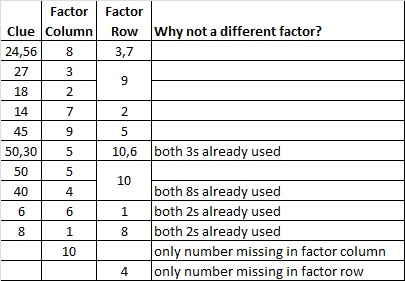# 53 and Animated Gif FIND THE FACTORS Level 5

I’m a mother and a grandmother. My most recent grandson, Oliver, was born three days ago on Tuesday.Steve Wilhite, the creator of gif graphics format, said, “Choosy mothers choose GIF.” I think that can apply to grandmothers, too.

I made my very first animated gif! You can see the factors from last week’s level 5 puzzle appear one by one right before your eyes! I set the gif at the slowest possible setting, but it still goes fairly fast. The puzzle is solved from start to finish in about 15 seconds.Here is this week’s level 5 puzzle. To solve it, write the numbers from 1 to 12 in the top row and again in the first column so that the numbers you write are the factors of the given clues. There is only one solution.This week’s puzzles are available in an excel file here. If you have a spreadsheet program on your computer, you can access it. If you enable editing in excel, you can type your answers directly onto the puzzle, and you can also easily print the puzzles.

Here are the factors from last week’s level 5 puzzle:How were those factors found? Look at the animated gif above or click here to see the puzzle being solved, or you can look at the chart below for a slightly different way to solve it.• 53 is a prime number.
• Prime factorization: 53 is prime.
• The exponent of prime number 53 is 1. Adding 1 to that exponent we get (1 + 1) = 2. Therefore 53 has exactly 2 factors.
• Factors of 53: 1, 53
• Factor pairs: 53 = 1 x 53
• 53 has no square factors that allow its square root to be simplified. √53 ≈ 7.2801How do we know that 53 is a prime number? If 53 were not a prime number, then it would be divisible by at least one prime number less than or equal to √53 ≈ 7.3. Since 53 cannot be divided evenly by 2, 3, 5, or 7, we know that 53 is a prime number.

1.wolfmarionette
•ivasallay